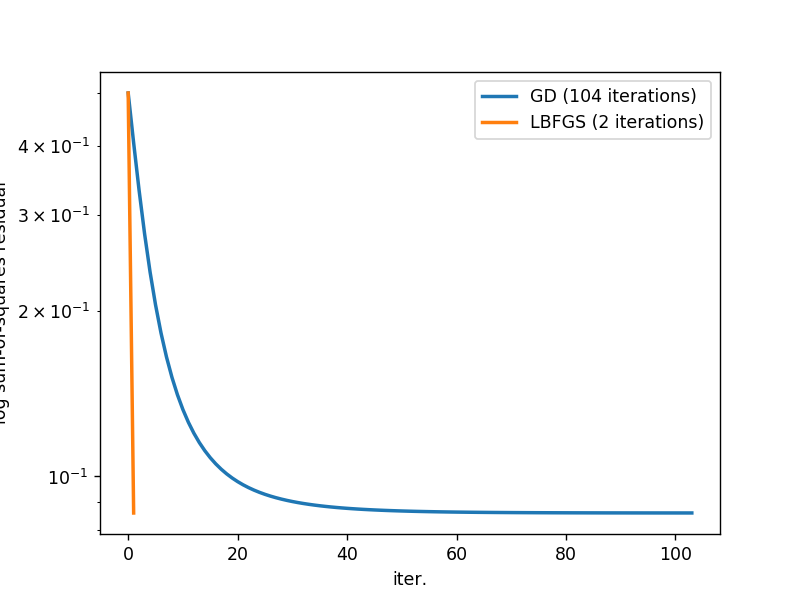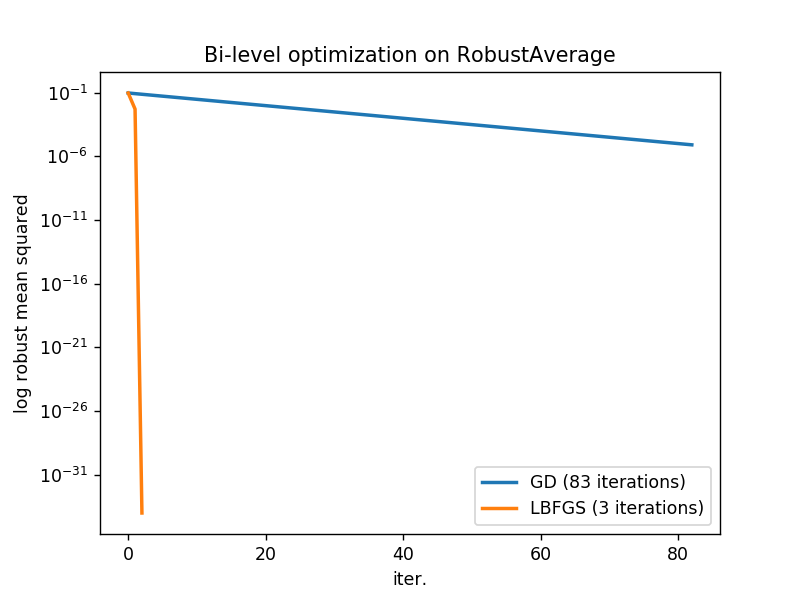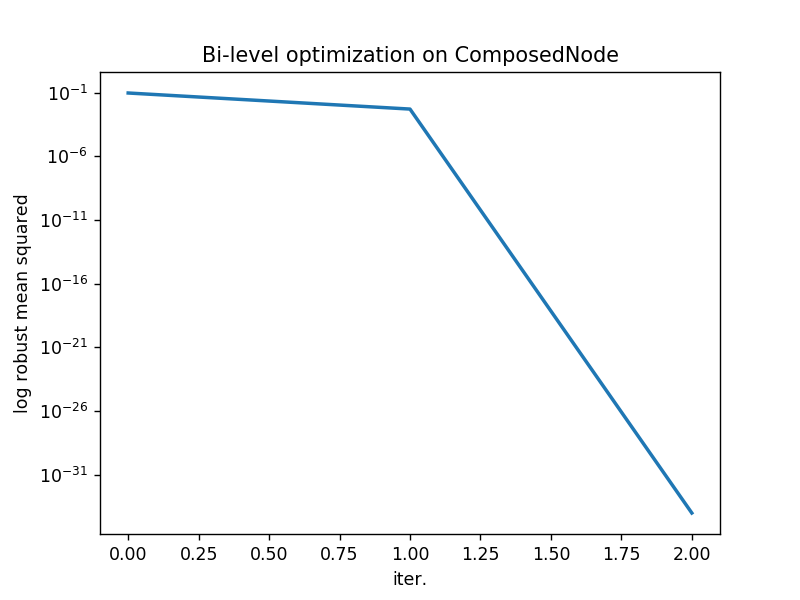# Worked Example of Bi-level Optimization¶

We present a worked example for bi-level optimimzation problems of the following form:

$$\begin{array}{ll} \text{minimize}_x & \frac{1}{2} \|y - y^\text{target}\|^2 \\ \text{subject to} & y \in \left\{ \begin{array}{ll} \text{argmin}_u & f(x, u)\\ \text{subject to} & h(x, u) = 0 \end{array} \right\} \end{array}$$

where the lower-level problem defines an equality constrained declarative node and the upper-level problem tries to learn the input $x$ such that the output of the declarative node matches some target value $y^\text{target}$ (or to get close to it in a least-squares sense).

In :
%matplotlib notebook

In :
import numpy as np
import scipy.optimize as opt
import matplotlib.pyplot as plt

import sys
sys.path.append("../")
from ddn.basic.node import *
from ddn.basic.sample_nodes import LinFcnOnUnitCircle
from ddn.basic.robust_nodes import RobustAverage


We begin by implementing a simple gradient descent procedure that computes

$$\text{D} J(y(x)) = \text{D}_Y J(y) \text{D} y(x)$$

where $J(y) = \frac{1}{2} \|y(x) - y^\text{target}\|^2$ and $\text{D}y$ is computed via implicity differentiation. Here $\text{D} J(y(x))$ is the derivative of $J$ with respect to $x$ (the independent variable) and $\text{D}_Y J(y)$ is the partial derivative of $J$ with respect to $y$. Our function is takes as input a deep declarative node, node, which exposes two methods that we will need:

• node.solve, which solves the (lower-level) optimization problem to produce $y$ given $x$, and
• node.gradient, which computes $\text{D}y$ given $x$ and optional $y$.
In :
def simpleGradientDescent(node, y_target, step_size=1.0e-3, tol=1.0e-6, max_iters=1000, x_init=None, verbose=False):
"""
An example of gradient descent for a simple bi-level optimization problem of the form:
minimize_{x} 0.5 * \|y - y_target\|^2
subject to y = argmin_u f(x, u) s.t. h(x, u) = 0

Returns the solution x found and learning curve (objective function J per iteration).
"""
assert y_target.shape == node.dim_y
x = x_init.copy() if x_init is not None else np.zeros((node.dim_x,))

J = lambda y : 0.5 * np.sum(np.square(y - y_target))
dJdy = lambda y : y - y_target

history = []
for i in range(max_iters):
# solve the lower-level problem and compute the upper-level objective
y, _ = node.solve(x)
history.append(J(y))
if verbose: print("{:5d}: {}".format(i, history[-1]))
if (len(history) > 2) and (history[-2] - history[-1]) < tol:
break

# compute the gradient of the upper-level objective with respect to x via the chain rule

# take a step in the negative gradient direction
x -= step_size * dJdx

return x, history


We can also implement a more sophisticated optimizer using L-BFGS:

In :
def lbfgs(node, y_target, max_iters=100, x_init=None, verbose=True):
"""
Example of using scipy.optimize to solve the problem via L-BFGS.
"""
assert y_target.shape == node.dim_y
x_start = x_init.copy() if x_init is not None else np.zeros((node.dim_x,))

def J(x):
y, _ = node.solve(x)
return 0.5 * np.sum(np.square(y - y_target))
def dJdx(x):
y, _ = node.solve(x)
return np.dot(y - y_target, node.gradient(x, y)).reshape((node.dim_x,))

history = [J(x_start)]
def callback(xk):
history.append(J(xk))

opts = {'maxiter': max_iters, 'disp': verbose}
result = opt.minimize(J, x_start, args=(), method='L-BFGS-B', jac=dJdx, options=opts, callback=callback)
return result.x, history


## Linear function on a circle¶

Let's test the code by trying to solve a simple bi-level optimization problem.

$$\begin{array}{ll} \text{minimize}_{x \in \mathbb{R}} & \frac{1}{2} \|y + (1, 1)\|^2 \\ \text{subject to} & y \in \left\{ \begin{array}{ll} \text{argmin}_{u \in \mathbb{R}^2} & (1, x)^T u\\ \text{subject to} & \|u\|^2 = 1 \end{array} \right\} \end{array}$$

By inspection, the solution to this problem is $x = 1$, which gives $y = -\left(\frac{1}{\sqrt{2}}, \frac{1}{\sqrt{2}}\right)$.

In :
node = LinFcnOnUnitCircle()
y_target = np.array([-1.0, -1.0])

x, history_gd = simpleGradientDescent(node, y_target, step_size=0.1)
y_star, _ = node.solve(x)
print("Gradient descent to give x = {} gives y = {}".format(x, y_star))

x, history_bfgs = lbfgs(node, y_target)
y_star, _ = node.solve(x)
print("L-BFGS finds x = {} to give y = {}".format(x, y_star))

plt.figure()
plt.semilogy(history_gd, lw=2)
plt.semilogy(history_bfgs, lw=2)
plt.xlabel("iter."); plt.ylabel("log sum-of-squares residual")
plt.legend(["GD ({} iterations)".format(len(history_gd)), "LBFGS ({} iterations)".format(len(history_bfgs))])
plt.show()

Gradient descent to give x = [0.99147837] gives y = [-0.71012602 -0.70407459]
L-BFGS finds x = [1.] to give y = [-0.70710678 -0.70710678]## Robust averaging¶

We now consider a more sophisticated example where we wish to optimize points to achieve a robust average of zero. We use the Huber penalty to estimate the robust average,

$$\begin{array}{ll} \text{minimize}_{x \in \mathbb{R}^n} & \frac{1}{2} y^2 \\ \text{subject to} & y \in \text{argmin}_{u \in \mathbb{R}} \sum_{i=1}^{n} \phi^\text{huber}(u - x_i; \alpha = 1) \end{array}$$

where

$$\phi^\text{huber}(z, \alpha) = \begin{cases} \frac{1}{2} z^2 & \text{if |z| \leq \alpha} \\ \alpha|z| - \frac{1}{2}\alpha^2 & \text{otherwise} \end{cases}$$

We start with randomly generated $n$-dimensional input, $x$.

In :
n = 10 # number of input points
node = RobustAverage(n, penalty='huber', alpha=1.0)
y_target = np.array([0.0])
x_init = np.random.rand(n)

x_init[np.random.randint(len(x_init))] += 100.0 * np.random.rand(1)

# show starting average
y_init, _ = node.solve(x_init)
print("Initial x = {}".format(x_init))
print("Mean of intial x is {}".format(np.mean(x_init)))
print("Robust mean of initial x is {}".format(y_init))
print()

x_gd, history_gd = simpleGradientDescent(node, y_target, step_size=0.5, x_init=x_init)
y_star, _ = node.solve(x_gd)
print("Gradient descent to give x = {}".format(x_gd))
print("Mean of x is {}".format(np.mean(x_gd)))
print("Robust mean of x is {}".format(y_star))
print()

# solver using L-BFGS
x_bfgs, history_bfgs = lbfgs(node, y_target, x_init=x_init)
y_star, _ = node.solve(x_bfgs)
print("L-BFGS finds x = {}".format(x_bfgs))
print("Mean of x is {}".format(np.mean(x_bfgs)))
print("Robust mean of x is {}".format(y_star))

# plot results
plt.figure()
plt.semilogy(history_gd, lw=2)
plt.semilogy(history_bfgs, lw=2)
plt.xlabel("iter."); plt.ylabel("log robust mean squared")
plt.title("Bi-level optimization on {}".format(type(node).__name__))
plt.legend(["GD ({} iterations)".format(len(history_gd)), "LBFGS ({} iterations)".format(len(history_bfgs))])
plt.show()

Initial x = [5.93542798e-02 3.55107942e-01 1.67732688e-01 6.99960114e+01
6.82992326e-01 5.96795968e-01 1.02817666e-02 3.19865546e-01
1.99863628e-01 5.22843473e-01]
Mean of intial x is 7.291084906338865
Robust mean of initial x is [0.43498196]

Gradient descent to give x = [-0.37161959 -0.07586593 -0.26324118 69.99601145  0.25201846  0.1658221
-0.4206921  -0.11110832 -0.23111024  0.0918696 ]
Mean of x is 6.903208424825077
Robust mean of x is [0.00400809]

L-BFGS finds x = [-0.37562768 -0.07987402 -0.26724927 69.99601145  0.24801037  0.16181401
-0.42470019 -0.11511641 -0.23511833  0.08786152]
Mean of x is 6.899601144547158
Robust mean of x is [-1.38777878e-17]## Simplification with composition¶

We can significantly simplify the code by using composition of declarative nodes, which automatically combines gradients. Here we compose the node that implements the lower-level problem, RobustAverage with a node that computes the squared error between its input and some target, SquaredErrorNode. Then bi-level optimization is achieved by minimizing the output of the composed node.

In :
from ddn.basic.composition import ComposedNode
from ddn.basic.sample_nodes import SquaredErrorNode

node = ComposedNode(RobustAverage(n, penalty='huber', alpha=1.0), SquaredErrorNode(1))

y_init, _ = node.solve(x_init)
history = [y_init]
result = opt.minimize(lambda xk: node.solve(xk), x_init, args=(), method='L-BFGS-B', jac=lambda xk: node.gradient(xk),
options={'maxiter': 1000, 'disp': False},
callback=lambda xk: history.append(node.solve(xk)))

# plot results
plt.figure()
plt.semilogy(history, lw=2)
plt.xlabel("iter."); plt.ylabel("log robust mean squared")
plt.title("Bi-level optimization on {}".format(type(node).__name__))
plt.show()In [ ]: